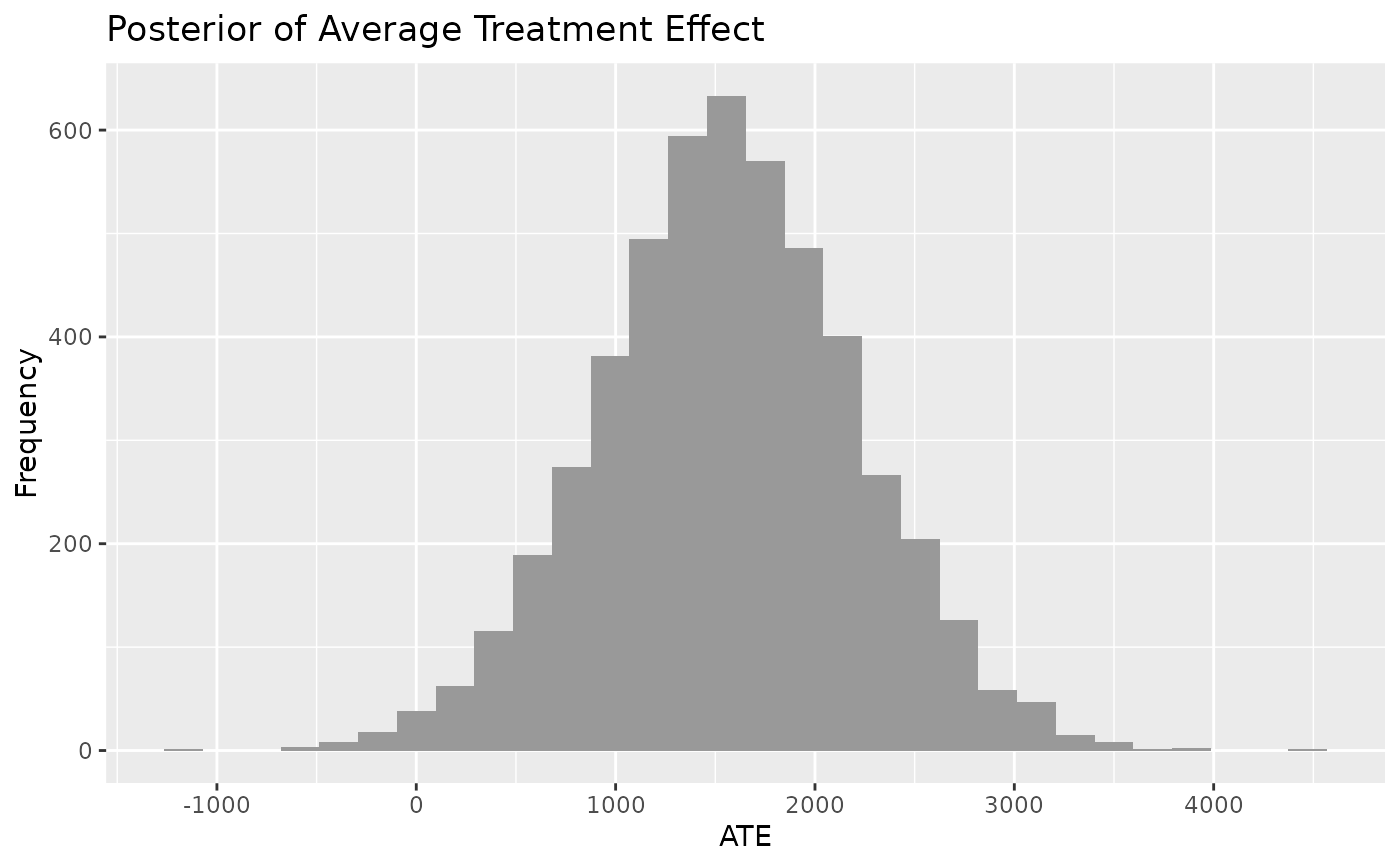Plot the conditional average treatment effect (CATE) of a 'bartCause' model. The conditional average treatment effect is derived from taking the difference between predictions for each individual under the control condition and under the treatment condition averaged over the population. Means of the CATE distribution will resemble SATE and PATE but the CATE distribution accounts for more uncertainty than SATE and less uncertainty than PATE.

## Usage

plot_CATE(
.model,
type = c("histogram", "density"),
ci_80 = FALSE,
ci_95 = FALSE,
reference = NULL,
.mean = FALSE,
.median = FALSE
)

## Arguments

.model

a model produced by bartCause::bartc()

type

histogram or density

ci_80

TRUE/FALSE. Show the 80% credible interval?

ci_95

TRUE/FALSE. Show the 95% credible interval?

reference

numeric. Show a vertical reference line at this value

.mean

TRUE/FALSE. Show the mean reference line

.median

TRUE/FALSE. Show the median reference line

ggplot object

## Author

George Perrett, Joseph Marlo

## Examples

# \donttest{
data(lalonde)
confounders <- c('age', 'educ', 'black', 'hisp', 'married', 'nodegr')
model_results <- bartCause::bartc(
response = lalonde[['re78']],
treatment = lalonde[['treat']],
confounders = as.matrix(lalonde[, confounders]),
estimand = 'ate',
commonSup.rule = 'none'
)
#> fitting treatment model via method 'bart'
#> fitting response model via method 'bart'
plot_CATE(model_results)
#> stat_bin() using bins = 30. Pick better value with binwidth.# }